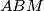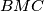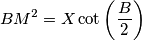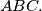### IMO Shortlist 1988 problem 30

Kvaliteta:
Avg: 0,0
Težina:
Avg: 0,0
A point$M$ is chosen on the side$AC$ of the triangle$ABC$ in such a way that the radii of the circles inscribed in the triangles$ABM$ and$BMC$ are equal. Prove thatwhere X is the area of triangle$ABC.$
Izvor: Međunarodna matematička olimpijada, shortlist 1988